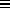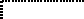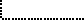Common Lisp the Language, 2nd EditionNext: Equality Predicates Up: Data Type Predicates Previous: General Type Predicates

## 6.2.2. Specific Data Type Predicates

The following predicates test for individual data types.

[Function]
null object

null is true if its argument is (), and otherwise is false. This is the same operation performed by the function not; however, not is normally used to invert a Boolean value, whereas null is normally used to test for an empty list. The programmer can therefore express intent by the choice of function name.

```(null x) == (typep x 'null) == (eq x '())
```

[Function]
symbolp object

symbolp is true if its argument is a symbol, and otherwise is false.

```(symbolp x) == (typep x 'symbol)
```

Compatibility note: The Interlisp equivalent of symbolp is called litatom.

[Function]
atom object

The predicate atom is true if its argument is not a cons, and otherwise is false. Note that (atom '()) is true, because ()nil.

```(atom x) == (typep x 'atom) == (not (typep x 'cons))
```

Compatibility note: In some Lisp dialects, notably Interlisp, only symbols and numbers are considered to be atoms; arrays and strings are considered to be neither atoms nor lists (conses).

[Function]
consp object

The predicate consp is true if its argument is a cons, and otherwise is false. Note that the empty list is not a cons, so (consp '()) == (consp 'nil) => nil.

```(consp x) == (typep x 'cons) == (not (typep x 'atom))
```

Compatibility note: Some Lisp implementations call this function pairp or listp. The name pairp was rejected for Common Lisp because it emphasizes too strongly the dotted-pair notion rather than the usual usage of conses in lists. On the other hand, listp too strongly implies that the cons is in fact part of a list, which after all it might not be; moreover, () is a list, though not a cons. The name consp seems to be the appropriate compromise.

[Function]
listp object

listp is true if its argument is a cons or the empty list (), and otherwise is false. It does not check for whether the list is a ``true list'' (one terminated by nil) or a ``dotted list'' (one terminated by a non-null atom).

```(listp x) == (typep x 'list) == (typep x '(or cons null))
```

[Function]
numberp object

numberp is true if its argument is any kind of number, and otherwise is false.

```(numberp x) == (typep x 'number)
```

[Function]
integerp object

integerp is true if its argument is an integer, and otherwise is false.

```(integerp x) == (typep x 'integer)
```

Compatibility note: In MacLisp this is called fixp. Users have been confused as to whether this meant integerp or fixnump, and so the name integerp has been adopted here.

[Function]
rationalp object

rationalp is true if its argument is a rational number (a ratio or an integer), and otherwise is false.

```(rationalp x) == (typep x 'rational)
```

[Function]
floatp object

floatp is true if its argument is a floating-point number, and otherwise is false.

```(floatp x) == (typep x 'float)
```[Function]
realp object

X3J13 voted in March 1989 (REAL-NUMBER-TYPE)   to add the function realp. realp is true if its argument is a real number, and otherwise is false.

```(realp x) == (typep x 'real)
```[Function]
complexp object

complexp is true if its argument is a complex number, and otherwise is false.

```(complexp x) == (typep x 'complex)
```

[Function]
characterp object

characterp is true if its argument is a character, and otherwise is false.

```(characterp x) == (typep x 'character)
```

[Function]
stringp object

stringp is true if its argument is a string, and otherwise is false.

```(stringp x) == (typep x 'string)
```

[Function]
bit-vector-p object

bit-vector-p is true if its argument is a bit-vector, and otherwise is false.

```(bit-vector-p x) == (typep x 'bit-vector)
```

[Function]
vectorp object

vectorp is true if its argument is a vector, and otherwise is false.

```(vectorp x) == (typep x 'vector)
```

[Function]
simple-vector-p object

vectorp is true if its argument is a simple general vector, and otherwise is false.

```(simple-vector-p x) == (typep x 'simple-vector)
```

[Function]
simple-string-p object

simple-string-p is true if its argument is a simple string, and otherwise is false.

```(simple-string-p x) == (typep x 'simple-string)
```

[Function]
simple-bit-vector-p object

simple-bit-vector-p is true if its argument is a simple bit-vector, and otherwise is false.

```(simple-bit-vector-p x) == (typep x 'simple-bit-vector)
```

[Function]
arrayp object

arrayp is true if its argument is an array, and otherwise is false.

```(arrayp x) == (typep x 'array)
```

[Function]
packagep object

packagep is true if its argument is a package, and otherwise is false.

```(packagep x) == (typep x 'package)
```

[Function]
functionp objectfunctionp is true if its argument is suitable for applying to arguments, using for example the funcall or apply function. Otherwise functionp is false.

functionp is always true of symbols, lists whose car is the symbol lambda, any value returned by the function special form, and any values returned by the function compile when the first argument is nil.X3J13 voted in June 1988 (FUNCTION-TYPE)   to define

```(functionp x) == (typep x 'function)
```

Because the vote also specifies that types cons and symbol are disjoint from the type function, this is an incompatible change; now functionp is in fact always false of symbols and lists.[Function]
compiled-function-p object

compiled-function-p is true if its argument is any compiled code object, and otherwise is false.

```(compiled-function-p x) == (typep x 'compiled-function)
```[Function]
commonp object

commonp is true if its argument is any standard Common Lisp data type, and otherwise is false.

```(commonp x) == (typep x 'common)
```X3J13 voted in March 1989 (COMMON-TYPE)   to remove the predicate commonp (and the type common) from the language.See also standard-char-p, string-char-p, streamp, random-state-p, readtablep, hash-table-p, and pathnamep.Next: Equality Predicates Up: Data Type Predicates Previous: General Type Predicates

AI.Repository@cs.cmu.edu